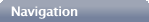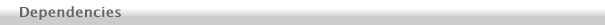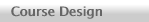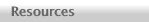Overview. I have tried to design this book to give instructors maximum flexibility in shaping a course that suits their students' needs. One does not have to go through this book sequentially from the first to the last chapter.General Section Dependencies. The flow chart that appears on the title page of each chapter shows how the major sections of the book depend on each other. For example, you can see from the chart that the Introduction, Flat Space, and Tensors sections (chapters 1 through 8) provide core material that every other section uses. I then recommend going on to the Schwarzschild Black Holes section because this will develop students' understanding of the metric and curved space before they encounter more math (and because black holes are fascinating!), but this is not necessary; in a short course focused on cosmology, for example, one could could go directly on to the Calculus of Curvature, Einstein Equation, and Cosmologiy sections. The chart also shows that the final three sections (Cosmology, Gravitational Waves, and Spinning Black Holes) are completely independent of each other and in principle could be presented in any order. However, all three of these sections require the Calculus of Curvature and Einstein Equation sections.Dependencies within the Schwarzschild Black Holes Section. One also does not have to go all the way through the Schwarzschild section. The last three chapters (on black holes) are only necessary if you plan to go through the last two chapters of the Spinning Black Holes section. One can easily omit the Deflection of Light chapter without loss of continuity. The Precession of the Perihelion chapter is necessary background for the Deflection of Light chapter, but otherwise could be omitted. The first two chapters are required for all of the other chapters in this section, and the Photon Orbits chapter introduces a mathematical technique that is used in selected homework problems throughout the remainder of the book, but techically is required only for the Deflection of Light and Black Hole Thermodynamics chapters.The Cosmology Section. In the Cosmology section, the first four chapters provide core material and should all be presented if this section is to be covered at all. The final two chapters, however, are completely optional; you can omit both or the last, as desired.The Gravitational Waves Section. While in principle it is possible to stop at after the first two chapters, I think that a discussion of gravitational wave energy and generation are important. I therefore recommend including all four chapters of this section if you discuss gravitational waves at all.The Spinning Black Holes Section. One might reasonably elect to present the Gravitomagnetism chapter alone in this section. If you are going to discuss Kerr black holes, one could stop either after the Kerr Particle Orbits chapter or Ergoregion and Horizon chapter. However, the chapters in this section do need to be discussed in sequence; one cannot easily drop a chapter from the middle.You can purchase a copy directly from the publisher here.New! Online Student Manual available here.Thomas A. Moore has been a professor in the Physics and Astronomy Department at Pomona College since 1987. He does theoretical research on detecting gravitational waves using LISA (now eLISA). Send him a message.Copyright © 2009, 2010, 2015 Thomas A. Moore